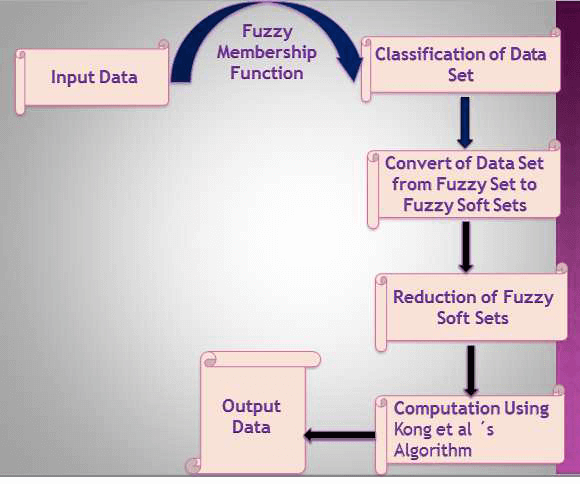Special lssues
Table of Content

# Application of Computer Tools in the Study of Mathematical Problems

Submission Deadline: 31 January 2023 (closed) Submit to Special Issue

### Guest Editors

Prof. Wenpeng Zhang, Northwest University, China
Prof. Jie Wu, French National Center for Scientific Research, France
Prof. Taekyun Kim, Kwangwoon University, South Korea

### Summary

It is well known that number theory and combinatorics are two very important research branches of pure mathematics, many mathematical problems are closely related to them, such as Riemann hypothesis, Waring problem, Goldbach conjecture, twin prime conjecture, etc. Number theory and combinatorics is mainly concerned with numbers, thus many problems on these two fields can be converted or expressed by computable formulae. Because of this, computer tools are widely applied to these problems. To be concrete, computer tools help to verify the accuracy of conjectures, improve the error term of the estimations of inequalities, calculate the numerical results of computational formulae and so on. Therefore, it is necessary to conduct in-depth research in these fields, in order to make some progress in the study of certain mathematical theoretical problems. This special issue will focus on the contents of the study of number theory and combinatorics, especially those pure mathematical problems that can be realized with the help of computer tools. We will convert such mathematical problems into the forms suitable for computers to solve. Of course, we are not limited to these.

The aim of this special issue is to study various recurrence sequences, combinatorial identities and the calculation of the power mean of exponential sums and character sums, and to obtain some precise calculation formulae for them. Due to the computational complexity of such problems, we try to solve them by using mathematical modeling or computer programming, in order to achieve better results.

Potential topics include but are not limited to the following:

· Recurrence sequences

· Various exponential sums and their power mean

· Combinatorial identities

· Mathematical modeling

· Computer programming

### Keywords

Recurrence sequences; combinatorial identities; exponential sums; character sums; mathematical modeling

### Published Papers

•Open Access

ARTICLE

On the Mean Value of High-Powers of a Special Character Sum Modulo a Prime

CMES-Computer Modeling in Engineering & Sciences, Vol.136, No.1, pp. 943-953, 2023, DOI:10.32604/cmes.2023.024363
（This article belongs to this Special Issue: Application of Computer Tools in the Study of Mathematical Problems)
Abstract In this paper, we use the elementary methods, the properties of Dirichlet character sums and the classical Gauss sums to study the estimation of the mean value of high-powers for a special character sum modulo a prime, and derive an exact computational formula. It can be conveniently programmed by the “Mathematica” software, by which we can get the exact results easily. More >

•Open Access

ARTICLE

Prediction System for Diagnosis and Detection of Coronavirus Disease-2019 (COVID-19): A Fuzzy-Soft Expert System

CMES-Computer Modeling in Engineering & Sciences, Vol.135, No.3, pp. 2715-2730, 2023, DOI:10.32604/cmes.2023.024755
（This article belongs to this Special Issue: Application of Computer Tools in the Study of Mathematical Problems)
Abstract In early December 2019, a new virus named “2019 novel coronavirus (2019-nCoV)” appeared in Wuhan, China. The disease quickly spread worldwide, resulting in the COVID-19 pandemic. In the current work, we will propose a novel fuzzy soft modal (i.e., fuzzy-soft expert system) for early detection of COVID-19. The main construction of the fuzzy-soft expert system consists of five portions. The exploratory study includes sixty patients (i.e., forty males and twenty females) with symptoms similar to COVID-19 in (Nanjing Chest Hospital, Department of Respiratory, China). The proposed fuzzy-soft expert system depended on five symptoms of COVID-19 (i.e., shortness of breath, sore… More >

Graphic Abstract